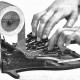Converting decimal numbers to fractions

Given that computer numbers have a finite precision, a calculation like 20/3 produces a result like 6.66667, which is slightly off. This begs the question: can we reverse the division operation, to make a routine that outputs “20/3” when it’s given the approximate value?

One trick is to take a large number as the denominator. For the numerator you multiply the floating point number with that large number and round the result. But this is suboptimal because we would get for example 66667/10000; never the expected 20/3. Can we do any better?

Yes, yes we can.

The best way to do this is with continued fractions, that is, fractions of the form:

$a_0+\cfrac{1}{a_1+\cfrac{1}{a_2+\cfrac{1}{\ddots}}}$

It turns out that continued fractions allow the construction of a sequence of rational numbers that approximate a given real number x really well. The first approximation is $$a_0$$, the next is $$a_0+1/a_1$$, the next $$a_0+\frac{1}{a_1+1/a_2}$$, and so on. The process to calculate the sequence of a’s is really simple: $$a_0$$ is just the integral part of x, floor(x). Then we calculate the inverse of the fractional part. The integral part of the result is $$a_1$$, and we can use the fractional part to repeat the process. A recurrence relation for the numerator and denominator allows us to update them in each step rather than having to calculate them using the above formula.

Why it works

Intuitively you can think of the process of calculating the a’s as lopping off as much of the error as possible in each step while limiting ourselves to inverses of integers: the error is the fractional part left over in each step. Indeed, for rational numbers eventually we would get fractional part of 0, meaning we have found the continued fraction representation of the number.

Formally, there’s a theorem that states that each approximation is nearer to the true value than any other fraction with a denominator less than that of the approximation. This means that the approximations produced by this process are as good as they get: other ratios are either longer or less precise.

Conclusions

Continued fractions have many interesting properties: Euler used them to prove that e is irrational. The continued fractions that eventually repeat are exactly the quadratic irrationals. The numerators and denominators produced by the process are coprime. The continued fraction representation of the golden ratio $$\varphi$$ is all 1’s, which means it’s the number that is worst approximated by continued fractions. In a sense this makes $$\varphi$$ the most irrational number of all.

You can find out more about continued fractions on the Wikipedia page.

1.Bjørn Nesby
Posted 2013/05/15 at 20:23 | Permalink

Wow, thank you!! The simplest implementation I’ve seen – using this in a project involving just intonation (the music theory), where practically everything is composed from ratios

2.Alyda
Posted 2016/03/09 at 18:28 | Permalink

This function can’t handle negative numbers. The demo returns -Infinity/Infinity.

3.Joni
Posted 2016/04/04 at 09:14 | Permalink

The easy fix is: if (x<0) return "-"+float2rat(-x).

The problem with negative numbers is that floor(x) always returns an integer less than or equal to x, so that -3.3 goes to -4. For this algorithm to work you would want a function that always rounds towards zero. You would also have to account for negative input in the loop termination condition.